伸展树

+关注继续查看

平衡查找树的一些限制：
1、平衡查找树每个节点都需要保存额外的信息。
2、难于实现，因此插入和删除操作复杂度高，且是潜在的错误点。
3、对于简单的输入，性能并没有什么提高。
平衡查找树可以考虑提高性能的地方：
1、平衡查找树在最差、平均和最坏情况下的时间复杂度在本质上是相同的。
2、对一个节点的访问，如果第二次访问的时间小于第一次访问，将是非常好的事情。
3、90-10法则。在实际情况中，90%的访问发生在10%的数据上。
4、处理好那90%的情况就很好了。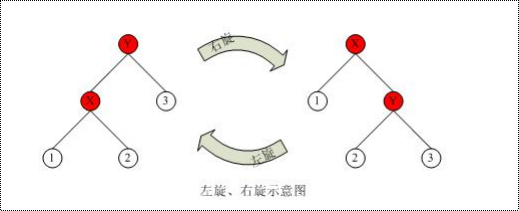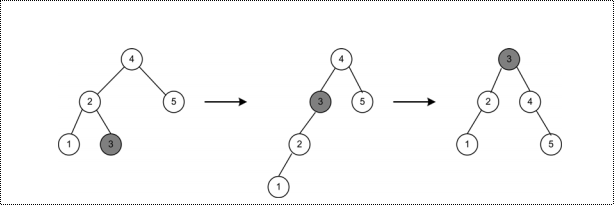图1

应用伸展（splaying）技术，可以得到对数均摊边界的时间复杂度。
在旋转的时候，可以分为三种情况：
1、zig情况。
X是查找路径上我们需要旋转的一个非根节点。
如果X的父节点是根，那么我们用下图所示的方法旋转X到根：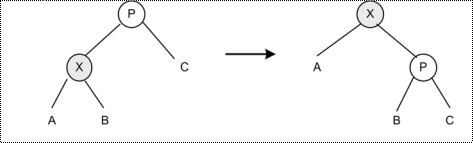图2
这和一个普通的单旋转相同。
2、zig-zag情况。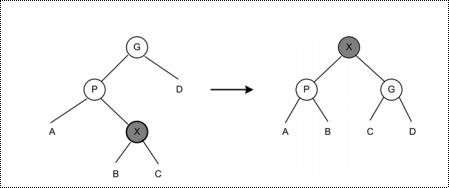图三
3、zig-zig情况。
这和前一个旋转不同。在这种情况中，X和P都是左子节点或右子节点。
先是P绕G右旋转，接着X绕P右旋转。
如图所示：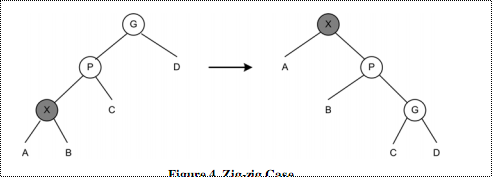图四
下面是splay的伪代码：
P(X) : 获得X的父节点，G(X) : 获得X的祖父节点（=P(P(X))）。
Function Buttom-up-splay:
Do
If X 是 P(X) 的左子结点 Then
If G(X) 为空 Then
X 绕 P（X）右旋
Else If P（X）是G（X）的左子结点
P(X) 绕G(X)右旋
X 绕P(X)右旋
Else
X绕P(X)右旋
X绕P(X)左旋 (P(X)和上面一句的不同，是原来的G(X))
Endif
Else If X 是 P(X) 的右子结点 Then
If G(X) 为空 Then
X 绕 P（X）左旋
Else If P（X）是G（X）的右子结点
P(X) 绕G(X)左旋
X 绕P(X)左旋
Else
X绕P(X)左旋
X绕P(X)右旋 (P(X)和上面一句的不同，是原来的G(X))
Endif
Endif
While (P(X) != NULL)
EndFunction
仔细分析zig-zag，可以发现，其实zig-zag就是两次zig。因此上面的代码可以简化：
Function Buttom-up-splay:
Do
If X 是 P(X) 的左子结点 Then
If P（X）是G（X）的左子结点
P(X) 绕G(X)右旋
Endif
X 绕P(X)右旋
Else If X 是 P(X) 的右子结点 Then
If P（X）是G（X）的右子结点
P(X) 绕G(X)左旋
Endif
X 绕P(X)左旋
Endif
While (P(X) != NULL)
EndFunction
下面是一个例子，旋转节点c到根上。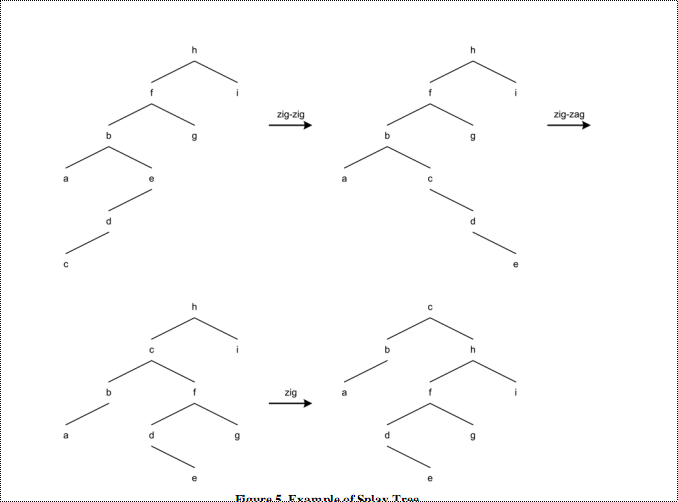图五

1、插入：
当一个节点插入时，伸展操作将执行。因此，新插入的节点在根上。
2、查找：
如果查找成功（找到），那么由于伸展操作，被查找的节点成为树的新根。

3、查找最大最小：
查找之后执行伸展。
4、删除最大最小：
a)删除最小：
首先执行查找最小的操作。

b)删除最大：

5、删除：
将要删除的节点移至根。
删除根，剩下两个子树L（左子树）和R（右子树）。
使用DeleteMax查找L的最大节点，此时，L的根没有右子树。
使R成为L的根的右子树。
如下图示：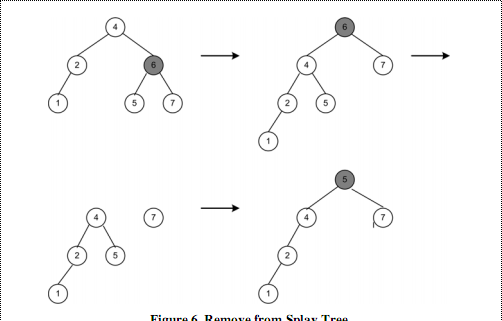图六

在自底向上的伸展树中，我们需要求一个节点的父节点和祖父节点，因此这种伸展树难以实现。因此，我们可以构建自顶向下的伸展树。
当我们沿着树向下搜索某个节点X的时候，我们将搜索路径上的节点及其子树移走。我们构建两棵临时的树──左树和右树。没有被移走的节点构成的树称作中树。在伸展操作的过程中：
1、当前节点X是中树的根。
2、左树L保存小于X的节点。
3、右树R保存大于X的节点。

1、zig：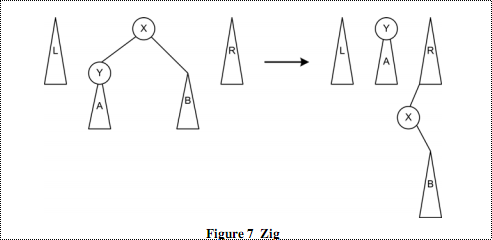图七
如上图，在搜索到X的时候，所查找的节点比X小，将Y旋转到中树的树根。旋转之后，X及其右子树被移动到右树上。很显然，右树上的节点都大于所要查找的节点。注意X被放置在右树的最小的位置，也就是X及其子树比原先的右树中所有的节点都要小。这是由于越是在路径前面被移动到右树的节点，其值越大。读者可以分析一下树的结构，原因很简单。
2、zig-zig: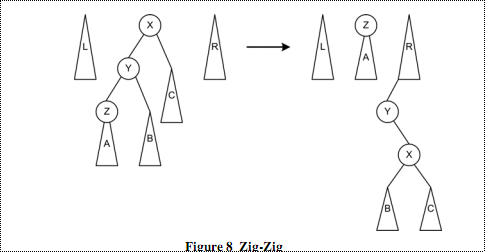图八
在这种情况下，所查找的节点在Z的子树中，也就是，所查找的节点比X和Y都小。所以要将X，Y及其右子树都移动到右树中。首先是Y绕X右旋，然后Z绕Y右旋，最后将Z的右子树（此时Z的右子节点为Y）移动到右树中。注意右树中挂载点的位置。
3、zig-zag: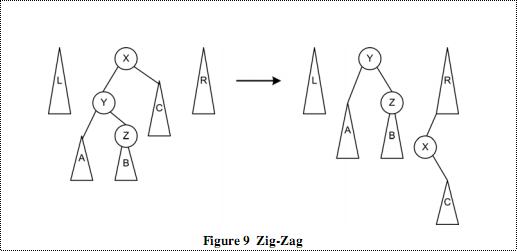图九
在这种情况中，首先将Y右旋到根。这和Zig的情况是一样的。然后变成上图右边所示的形状。接着，对Z进行左旋，将Y及其左子树移动到左树上。这样，这种情况就被分成了两个Zig情况。这样，在编程的时候就会简化，但是操作的数目增加（相当于两次Zig情况）。
最后，在查找到节点后，将三棵树合并。如图：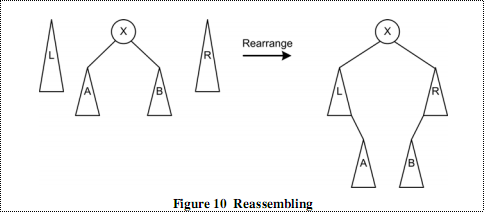图十
将中树的左右子树分别连接到左树的右子树和右树的左子树上。将左右树作为X的左右子树。重新最成了一所查找的节点为根的树。
下面给出伪代码：
右连接：将当前根及其右子树连接到右树上。左子结点作为新根。
左连接：将当前根及其左子树连接到左树上。右子结点作为新根。
T ： 当前的根节点。
Function Top-Down-Splay
Do
If X 小于 T Then
If X 等于 T 的左子结点 Then
右连接
ElseIf X 小于 T 的左子结点 Then
T的左子节点绕T右旋
右连接
Else X大于 T 的左子结点 Then
右连接
左连接
EndIf
ElseIf X大于 T Then
IF X 等于 T 的右子结点 Then
左连接
ElseIf X 大于 T 的右子结点 Then
T的右子节点绕T左旋
左连接
Else X小于 T 的右子结点‘ Then
左连接
右连接
EndIf
EndIf
While  ！(找到 X或遇到空节点)
组合左中右树
EndFunction

同样，上面的三种情况也可以简化：
Function Top-Down-Splay
Do
If X 小于 T Then
If X 小于 T 的左孩子 Then
T的左子节点绕T右旋
EndIf
右连接
Else If X大于 T Then
If X 大于 T 的右孩子 Then
T的右子节点绕T左旋
EndIf

EndIf
While  !(找到 X或遇到空节点)

EndFuntion

下面是一个查找节点19的例子：
在例子中，树中并没有节点19,最后，距离节点最近的节点18被旋转到了根作为新的根。节点20也是距离节点19最近的节点，但是节点20没有成为新根，这和节点20在原来树中的位置有关系。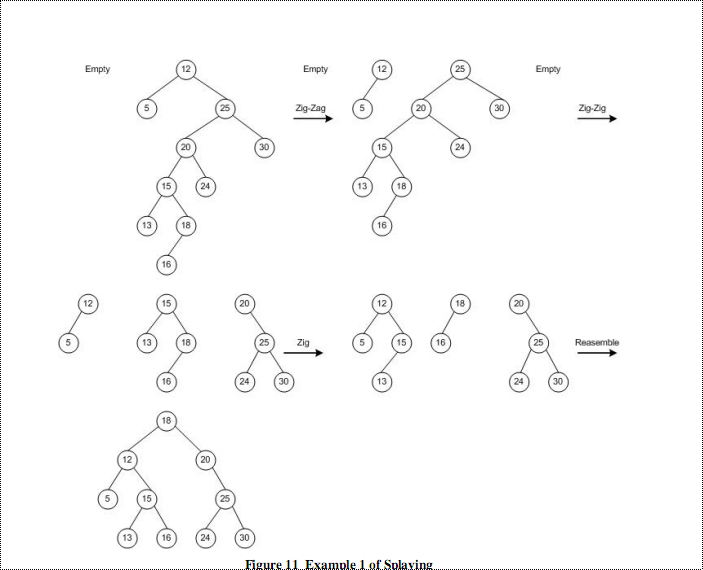这个例子是查找节点c：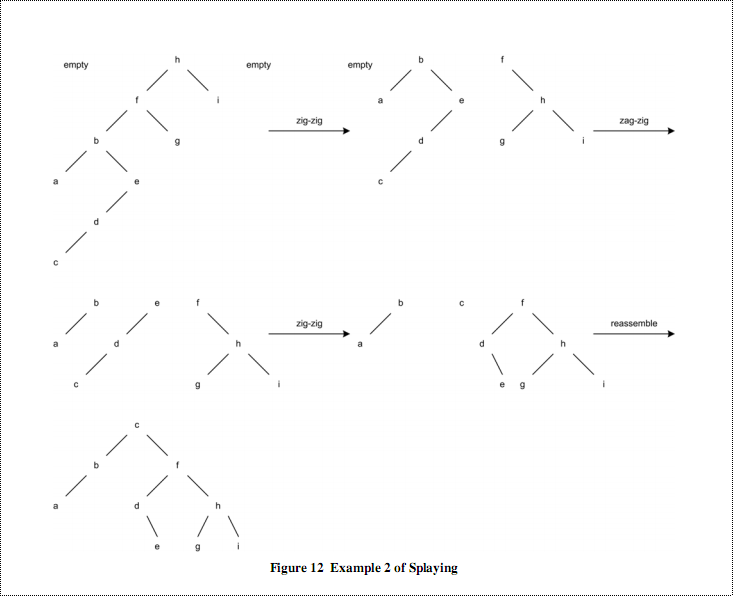/*
An implementation of top-down splaying
D. Sleator <sleator@cs.cmu.edu>
March 1992
*/
#include <stdlib.h>
#include <stdio.h>
int size;  /* number of nodes in the tree */
/* Not actually needed for any of the operations */
typedef struct tree_node Tree;
struct tree_node
{
Tree * left, * right;
int item;
};

Tree * splay (int i, Tree * t)
{
/* Simple top down splay, not requiring i to be in the tree t.  */
/* What it does is described above.                             */
Tree N, *l, *r, *y;
if (t == NULL)
return t;
N.left = N.right = NULL;
l = r = &N;
for (;;)
{
if (i < t->item)
{
if (t->left == NULL)
{
break;
}
if (i < t->left->item)
{
y = t->left;                           /* rotate right */
t->left = y->right;
y->right = t;
t = y;
if (t->left == NULL)
{
break;
}
}
r->left = t;                               /* link right */
r = t;
t = t->left;
}
else if (i > t->item)
{
if (t->right == NULL)
{
break;
}
if (i > t->right->item)
{
y = t->right;                          /* rotate left */
t->right = y->left;
y->left = t;
t = y;
if (t->right == NULL)
{
break;
}
}
l->right = t;                              /* link left */
l = t;
t = t->right;
}
else
{
break;
}
}
l->right = t->left;                                /* assemble */
r->left = t->right;
t->left = N.right;
t->right = N.left;
return t;
}
/* Here is how sedgewick would have written this.                    */
/* It does the same thing.                                           */
Tree * sedgewickized_splay (int i, Tree * t)
{
Tree N, *l, *r, *y;
if (t == NULL)
{
return t;
}
N.left = N.right = NULL;
l = r = &N;
for (;;)
{
if (i < t->item)
{
if (t->left != NULL && i < t->left->item)
{
y = t->left;
t->left = y->right;
y->right = t;
t = y;
}
if (t->left == NULL)
{
break;
}
r->left = t;
r = t;
t = t->left;
}
else if (i > t->item)
{
if (t->right != NULL && i > t->right->item)
{
y = t->right;
t->right = y->left;
y->left = t;
t = y;
}
if (t->right == NULL)
{
break;
}
l->right = t;
l = t;
t = t->right;
}
else
{
break;
}
}
l->right=t->left;
r->left=t->right;
t->left=N.right;
t->right=N.left;
return t;
}

Tree * insert(int i, Tree * t)
{
/* Insert i into the tree t, unless it's already there.    */
/* Return a pointer to the resulting tree.                 */
Tree * new1;

new1 = (Tree *) malloc (sizeof (Tree));
if (new1 == NULL)
{
printf("Ran out of space\n");
exit(1);
}
new1->item = i;
if (t == NULL)
{
new1->left = new1->right = NULL;
size = 1;
return new1;
}
t = splay(i,t);
if (i < t->item)
{
new1->left = t->left;
new1->right = t;
t->left = NULL;
size ++;
return new1;
}
else if (i > t->item)
{
new1->right = t->right;
new1->left = t;
t->right = NULL;
size++;
return new1;
}
else
{
/* We get here if it's already in the tree */
/* Don't add it again                      */
free(new1);
return t;
}
}

Tree * delete1(int i, Tree * t)
{
/* Deletes i from the tree if it's there.               */
/* Return a pointer to the resulting tree.              */
Tree * x;
if (t==NULL)
{
return NULL;
}
t = splay(i,t);
if (i == t->item)
{               /* found it */
if (t->left == NULL)
{
x = t->right;
}
else
{
x = splay(i, t->left);
x->right = t->right;
}
size--;
free(t);
return x;
}
return t;                         /* It wasn't there */
}

int main(int argv, char *argc[])
{
/* A sample use of these functions.  Start with the empty tree,         */
/* insert some stuff into it, and then delete it                        */
Tree * root;
int i;
root = NULL;              /* the empty tree */
size = 0;
for (i = 0; i < 1024; i++)
{
root = insert((541*i) & (1023), root);
}
printf("size = %d\n", size);
for (i = 0; i < 1024; i++)
{
root = delete1((541*i) & (1023), root);
}
printf("size = %d\n", size);
}26492 08480 02777 010919 010281 012149 0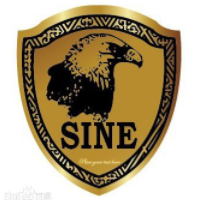windows server 2008阿里云ECS服务器安全设置

8719 07276 053717 021636 0

916

0

《2021云上架构与运维峰会演讲合集》

《零基础CSS入门教程》

《零基础HTML入门教程》